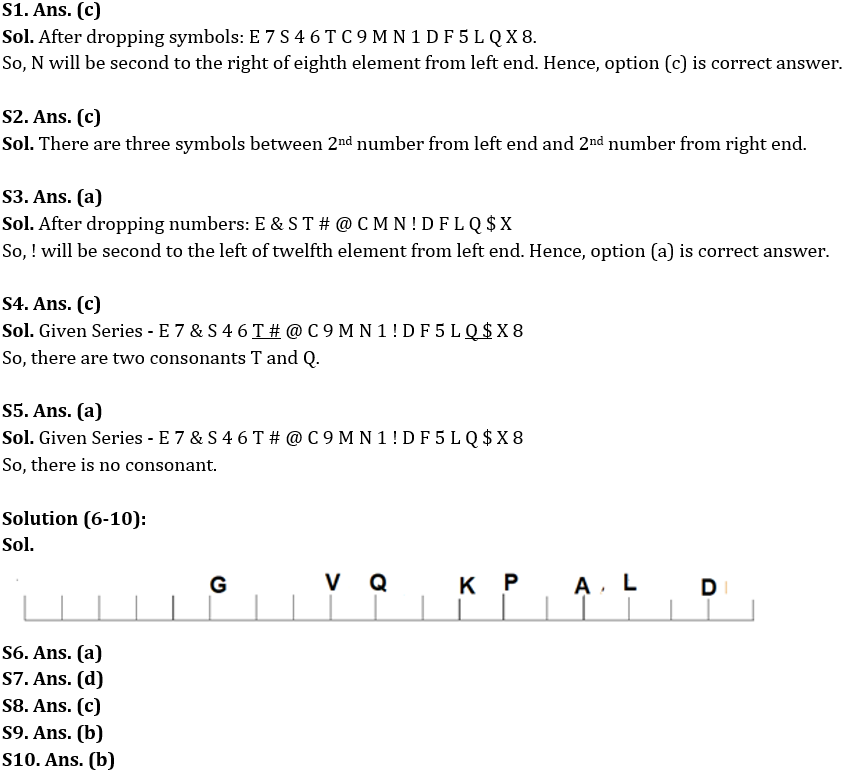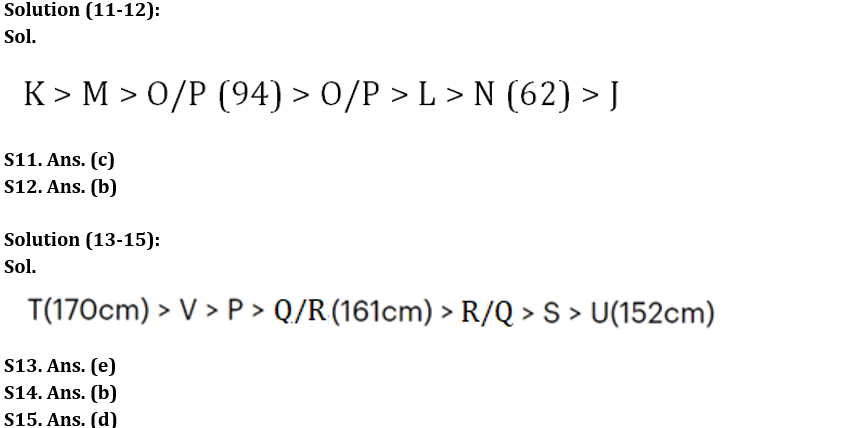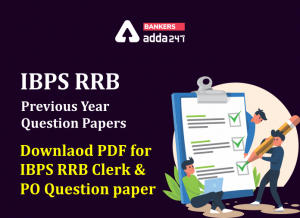Latest Banking jobs   »

# Reasoning Ability Quiz For IBPS RRB PO Prelims 2022- 29th June

Directions (1-5): study the following series carefully & answer the questions:

E 7 & S 4 6 T # @ C 9 M N 1 ! D F 5 L Q \$ X 8

Q1. If all the symbols are dropped, then which element will be second to the right of eighth element from left end?
(a) 9
(b) M
(c) N
(d) 1
(e) D

Q2. How many symbols lies between 2nd number from left end and 2nd number from right end?
(a) 5
(b) 4
(c) 3
(d) 2
(e) 1

Q3. If all the numbers are dropped, then which element will be second to the left of twelfth element from left end?
(a) !
(b) 1
(c) D
(d) 5
(e) None of the above

Q4. How many such consonants are in the above arrangement, each of which is immediately preceded by a vowel or immediately followed by a symbol but not both?
(a) None
(b) One
(c) Two
(d) Three
(e) Four

Q5. How many such consonants are in the above arrangement, each of which is immediately followed by a vowel but not immediately preceded by a number?
(a) None
(b) Two
(c) Three
(d) Four
(e) Five

Directions (6-10): Answer the questions based on the information given below:
Certain number of persons sits in a straight row facing north. V doesn’t sit adjacent to L. Five persons sit between K and D. A sits exactly between K and D. Five persons sit between A and V. L sits 4th to the right of K. D sits 2nd from one of the extreme ends. Two persons sit between V and G. Number of persons to the right of G is two more than those to the left of K. P sits 3rd to the right of Q, who sits exactly in the middle of the row.

Q6. Who sits adjacent to P?
(a) K
(b) A
(c) None of these
(d) L
(e) G

Q7. How many persons sit to the left of G?
(a) Six
(b) Nine
(c) Four
(d) Five
(e) None of these

Q8. How many persons sit in the row?
(a) 21
(b) 17
(c) 19
(d) 15
(e) None of these

Q9. Who among the following doesn’t sit to the left of Q?
I. V
II. K
III. G
(a) Only I
(b) Only II
(c) Only III
(d) Only I and II
(e) Only II and III

Q10. What is the position of Q with respect to K?
(a) 3rd to the left
(b) 2nd to the left
(c) 4th to the right
(d) 2nd to the right
(e) None of these

Directions (11-12): Answer the questions based on the information given below:
Seven students J, K, L, M, N, O and P scored different marks in an examination. L scored more than only J and N. M scored less than only K. N does not score the least. The one who scored the third-highest marks, scored 94 marks and N scored 62 marks. The student who scored the maximum marks among all, scored 16 marks more than O’s marks.

Q11. If O scored 94 marks, then what will be sum of the marks scored by N and K?
(a) 175
(b) 176
(c) 172
(d) 185
(e) None of these

Q12. What may be possible marks scored by L, if O and P scored 94 and 80 marks respectively?
(a) 95
(b) 70
(c) 85
(d) 90
(e) 61

Directions (13-15): Answer the following question based on the information below.
Seven people, P, Q, R, S, T, U and V have different heights. P is taller than Q and R but shorter than T. S is taller than U but shorter than both R and Q. V is taller than P but is not the tallest. The height of the tallest person is 170cm. The height of the shortest person is 152 cm. The height of fourth tallest person is the average of tallest and shortest person.

Q13. How many people are taller than Q?
(a) One
(b) Three
(c) Two
(d) Four
(e) Either three or four

Q14. If R is shorter than Q, then what can be the possible height of R?
(a) 163cm
(b)157cm
(c) 164cm
(d) 174cm
(e) 160cm

Q15. Who among the following people is 2nd tallest?
(a) T
(b) P
(c) Either T or V
(d) V
(e) None of these

Solutions#### Congratulations!Incorrect details? Fill the form again here

•Reasoning Ability Quiz For IBPS RRB PO C...
•Quantitative Aptitude Quiz For IBPS RRB ...
•Reasoning Ability Quiz For IBPS RRB PO C...
•Quantitative Aptitude Quiz For IBPS RRB ...
•IBPS RRB Previous Year Question Paper PD...
•Quantitative Aptitude Quiz For IBPS RRB ...